# Parallel scalability

This code can be used to test the scalability of common operations (traversal, restriction, boundary conditions etc…) and their combinations, in particular the multigrid Poisson solver.

#include "poisson.h"
#include "utils.h"
#include "check_restriction.h"

scalar a[], b[];

static void mpi_print (timer t, int i, size_t tnc,
const char * name)
{
double mpi[npe()];
timing s = timer_timing (t, i, tnc, mpi);

if (pid() == 0) {

s.min/i, s.avg/i, s.max/i are the minimum, average and maximum *times spent in communication routines.

    printf ("%d %g %g %g %s %g %g %g %.2g%% %ld %ld ",
npe(), s.cpu/i, s.real/i, s.speed, name, s.min/i, s.avg/i, s.max/i,
100.*s.avg/s.real, s.mem, s.tnc);

We also output the times spent in communication routines for each process.

    for (int j = 0; j < npe(); j++)
printf (" %g", mpi[j]/i);
printf ("\n");
}

MPI_Barrier (MPI_COMM_WORLD);
}

int main (int argc, char * argv[])
{
int maxlevel = argc > 1 ? atoi(argv) : 6;
int minlevel = argc > 2 ? atoi(argv) : 5;
timer t;

init_grid (1);
refine (level <= minlevel*(1. - sqrt(sq((x - 0.5) - 0.1) +
sq((y - 0.5)- 0.1))));

foreach()
a[] = b[] = 0.;
boundary ({a, b});
poisson (a, b); // to force allocation of extra fields

MPI_Barrier (MPI_COMM_WORLD);
t = timer_start();
refine (level <= maxlevel*(1. - sqrt(sq((x - 0.5) - 0.1) +
sq((y - 0.5) - 0.1))));
check_restriction (a);

size_t tnc = 0;
foreach(reduction(+:tnc))
tnc++;
mpi_print (t, 1, tnc, "refine");

if (npe() <= 8)
debug_mpi (qerr);

int nloops, i;

We fill a with a simple function.

  MPI_Barrier (MPI_COMM_WORLD);
t = timer_start();
i = nloops = npe();
while (i--) {
foreach()
a[] = cos(pi*x)*cos(pi*y);
#if 0
boundary ({a});
#else
prof_start ("barrier");
MPI_Barrier (MPI_COMM_WORLD);
prof_stop();
#endif
}
mpi_print (t, nloops, tnc*nloops, "cos");
boundary ({a});

Here we compute \displaystyle b = \nabla^2 a using a 5-points Laplacian operator.

  MPI_Barrier (MPI_COMM_WORLD);
t = timer_start();
i = nloops = npe();
while (i--) {
foreach()
b[] = (a[0,1] + a[1,0] + a[0,-1] + a[-1,0] - 4.*a[])/sq(Delta);
boundary ({b});
}
mpi_print (t, nloops, tnc*nloops, "laplacian");

Something simpler: the sum of a over the entire mesh.

  MPI_Barrier (MPI_COMM_WORLD);
t = timer_start();
i = nloops = npe();
double sum = 0.;
while (i--) {
sum = 0.;
foreach(reduction(+:sum))
sum += sq(Delta)*b[];
}
mpi_print (t, nloops, tnc*nloops, "sum");
fprintf (qerr, "sum: %g\n", sum);

The restriction operator.

  MPI_Barrier (MPI_COMM_WORLD);
t = timer_start();
i = nloops = 1;
while (i--) {
boundary ({b});
restriction ({b});
}
mpi_print (t, nloops, tnc*nloops, "restriction");

And finally the Poisson solver.

  MPI_Barrier (MPI_COMM_WORLD);
t = timer_start();
i = nloops = 1;
TOLERANCE = HUGE;
while (i--)
poisson (a, b);
mpi_print (t, nloops, tnc*nloops, "poisson");

scalar e[];
foreach()
e[] = a[] - cos(pi*x)*cos(pi*y);
fprintf (qerr, "error: %g\n", normf(e).max);
//  output_ppm (e, file = "error.png", n = 512);
//  assert (normf(e).max < 0.4);

int n = 0;
foreach()
n++;
int nmin = n, nmax = n;
mpi_all_reduce (nmin, MPI_INT, MPI_MIN);
mpi_all_reduce (nmax, MPI_INT, MPI_MAX);
fprintf (qerr, "balance %d %d\n", nmin, nmax);
}

## How to run on Curie

This test is run on Curie. The C99 source code is generated on a system with qcc installed and then copied to Curie using something like

qcc -source mpi-laplacian.c
scp _mpi-laplacian.c popinets@curie-fr.ccc.cea.fr:

On Curie the following script (run.sh) is used to compile and run the code

#!/bin/bash
#MSUB -r mpi-laplacian
#MSUB -n 32
#MSUB -T 600
####MSUB -Q test
#MSUB -o basilisk_%I.out
#MSUB -e basilisk_%I.log
#MSUB -q standard
#MSUB -A gen7325
#MSUB -w

LEVEL=10

set -x
cd ${BRIDGE_MSUB_PWD} mpicc -O2 -Wall -std=c99 -D_MPI=1 _mpi-laplacian.c -o mpi-laplacian -lm rm -f trace-* ccc_mprun -n${BRIDGE_MSUB_NPROC} ./mpi-laplacian ${LEVEL} 8 \ 2> log-${LEVEL}-${BRIDGE_MSUB_NPROC} > out-${LEVEL}-${BRIDGE_MSUB_NPROC} LEVEL is varied from 10 (~1 million grid points) to 15 (~1 billion grid points) and the number of processes up to 16384 using something like for i in 16 32 64 128 256 512 1024 2048 4096 8192 16384; do ccc_msub -n$i run.sh;
done

The results can then be collected using

tar czvf curie.tgz out-*-*

## Results on Curie

The memory usage per core is given below. The curves are a model (see mpi-laplacian.plot for details). The increase for a large number of cores corresponds to the memory overhead of communication buffers etc…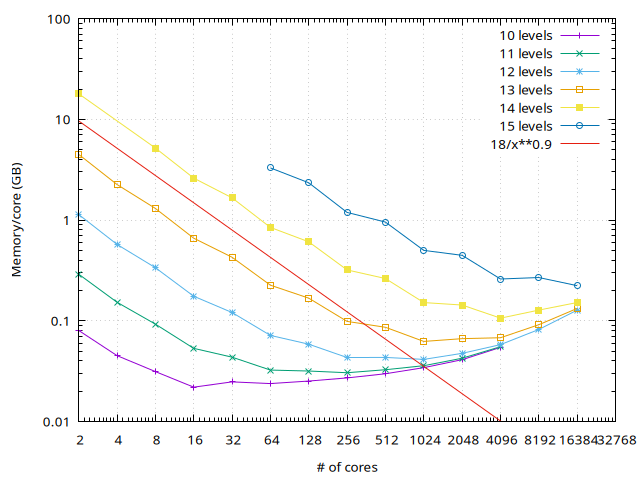Memory usage on Curie

The wall-clock time for one iteration of the multigrid Poisson solver is given below. The red lines are a model of strong scaling. For a low enough number of cores, close to perfect scaling is obtained with a best fit computation speed close to 1.5 million grid points/core.

The pink lines connect points corresponding with weak (or iso-granular) scaling i.e. quadrupling both the computation size and the number of cores. The ideal weak scaling would give horizontal lines (i.e. constant computation time for proportionally-increasing problem sizes and number of cores).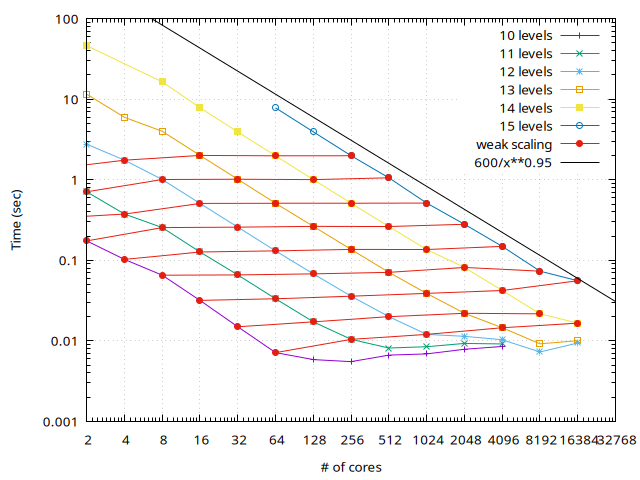Wall-clock time on Curie for the Poisson solver

The time spent in communication routines is illustrated below, together with the model (corresponding to the communication part of the total time in the previous figure).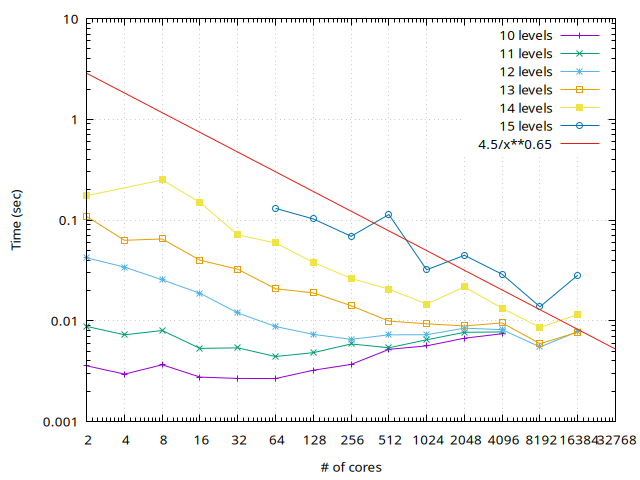Communication time on Curie for the Poisson solver

Similar results are obtained for a pure Laplacian with a best fit speed of order 25 million grid points/core. This speed is larger than for the Poisson solver and hence does not scale as well.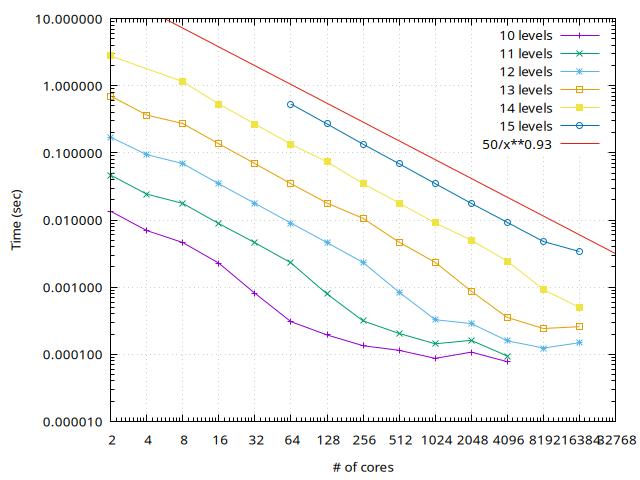Wall-clock time on Curie for the LaplacianCommunication time on Curie for the Laplacian

Similarly, the pure restriction operator scales quite well, with a best fit speed of around 30 million grid points/core.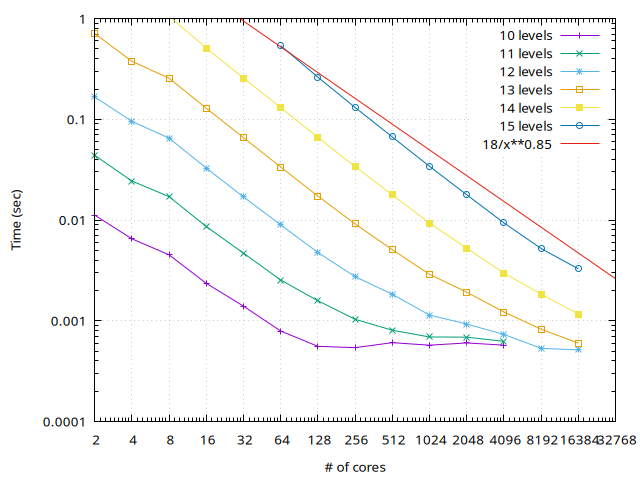Wall-clock time on Curie for the restriction operatorCommunication time on Curie for the restriction operator